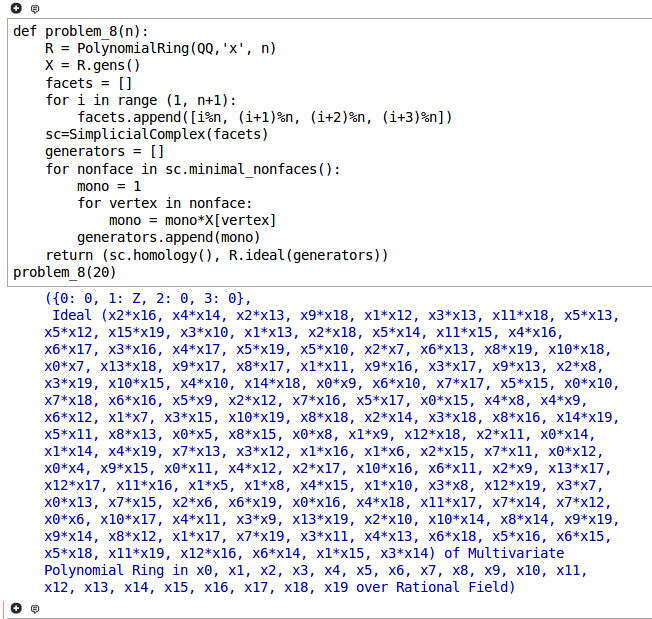# Problem 8

Consider the three-dimensional simplicial complex on $$n$$ vertices having the $$n$$ facets $$\{i,i+1,i+2,i+3\}$$, for $$i=1,2,\ldots,n$$, cyclically rotated. For $$n \leq 20$$, compute the Stanley-Reisner ideals and all homology groups.

## Solution

Here is a solution in Sage:

def problem_8(n):
R = PolynomialRing(QQ,'x', n)
X = R.gens()
facets = []
for i in range (1, n+1):
facets.append([i%n, (i+1)%n, (i+2)%n, (i+3)%n])
sc=SimplicialComplex(facets)
generators = []
for nonface in sc.minimal_nonfaces():
mono = 1
for vertex in nonface:
mono = mono*X[vertex]
generators.append(mono)
return (sc.homology(), R.ideal(generators))

problem_8(20)